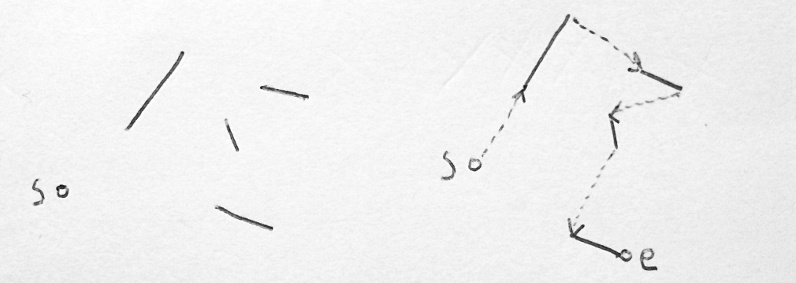back to lofibucket.com

When optimizing the path of the electron beam of an oscilloscope in X-Y mode one wants to make the path as short as possible to increase the number of shapes visible on screen simultaneously.

Assuming we have the case where all shapes are made of straight line segments, it’s pretty easy to see why the problem of finding the shortest path is NP-hard. This means there’s no fast algorithm for it that always produces the optimal result.

## Solving the Traveling Salesman Problem

So we have a bunch of two-dimensional line segments. The line segments are represented as pairs of 2D points (a, b) where a and b are the start and end point of the line segment, respectively. The problem is to find the shortest path that visits every line exactly once. It doesn’t matter which direction we traverse a line segment. We are also given a distinct start point s where to begin, but it doesn’t matter where the path ends. Let’s call this the shortest line segment path problem 1 just to make things clearer later on.Starting point s and some line segments and a path that visits all line segments and ends in point e.

We are going to use a reduction proof to show that this problem is NP-hard. In a reduction proof we translate some existing hard problem into an instance of our new problem. Then we know that also the new problem must be NP-hard. Concretely, we are going to show that if you know how to solve the shortest line segment path problem, you can also solve the Traveling Salesman Problem (TSP) with it.

In the Traveling Salesman Problem you need to find the shortest path that visits n cities and then returns to the city where the trip started. We are going relax the latter constraint and say we don’t really care where the path ends. This variant of the problem is still NP-hard. 2

Assume that we know how to optimally solve the shortest line segment problem. So we go and create a single line segment for each of the n cities. Each line segment consists of a pair of 2D points as defined above. The trick here that the start and end points are identical i.e. each line segment is a pair (c, c) where c is the position of the city. They are still totally legit line segments and we can feed them into our solving algorithm that returns the shortest path visiting each segment. We now have a shortest path that visits every city (line segment), so we solved TSP and therefore the shortest line segment problem must be NP-hard.

It’s important to note the direction of the reduction here, because it isn’t enough to show that we can solve the line segment path problem using TSP. That would just tell us that line segment path problem isn’t harder than TSP, and we wanted to know if it’s at least as hard as TSP.

1. I tried to look up this problem in the literature but couldn’t find exactly this formulation. It’s somewhat related to the Chinese postman problem and finding a minimum Eulerian path but not either of them directly apply.

2. You can call it the divorced salesman problem if you wish. Jokes aside, turns out unhappy drivers are an algorithmic problem too.